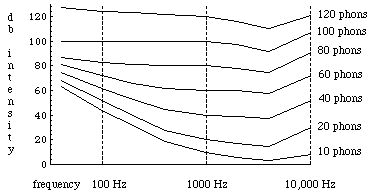# Physics of Hearing

There are two essential issues concerning the physics of hearing. Both involve understanding subjective phenomena: loudness and "frequency response". We must distinguish between loudness and intensity since intensity is a function purely of the power output of and the distance from the source (see Section A), while loudness is a sensation which depends greatly on a number of variables, including the sound frequencies being heard. Frequency response is the range of frequencies which a given individual can hear.

We measure sound intensity in terms of "decibels". The decibel is a "relative unit" which is actually dimensionless, comparing a given sound to a standard intensity which represents the smallest audible sound:

db = 10 log 10 ( I / I 0),

(I 0 is 10 - 16 W / cm 2 at 1000 Hz). 0 db thus represents the softest audible sound, while 80 db (ie., moderately loud music) represents an intensity which is one hundred million times greater. Since intensity is power per unit area, we can change the measured db by either changing the power or changing the distance from the source. For instance, if we find the 80 db too loud and prefer 40 db instead, we need either reduce the power by a factor of 10,000:

D db = 10 (log 10 (I f / I 0) - log 10 (I i / I 0))

= 10 log 10 (I f / I i)

= 10 log 10 (1 / 10,000)

= - 40 db

(i and f refer to initial and final), or move one hundred times further away:

D db = 10 log 10 ((P f / 4 p r f2) / (P i / 4 p r i2))

= 10 log 10 (ri2 / r f2))

= 10 log 10 (1 / (1002))

= - 40 db.

Here we have assumed that the sound is omnidirectional, and so takes the form of a spherical wave with its intensity spread equally over the expanding spherical wave "front".

So far we have only expressed the objective quantity intensity in relation to a standard subjective threshold. The loudness of a sound depends not only on the intensity, but also on the frequency and the sensitivity of the individual ear:

1. the cochlea has natural modes which are easier to excite (unrolled, it is approximately cylindrical; the natural modes are those which satisfy the closed - closed boundary conditions);
2. different frequencies excite different modes on the tympanic membrane: each mode has a different effective area, with corresponding different amplitudes;
3. reflected energy inside the ear, as well as viscous losses, are a function of energy; energy often varies with frequency;
4. the overall ear mechanism is stiffer at low frequency, whereas at higher frequencies, inertia of the components is the limiting factor: losses are least (they are only frictional) between 1000 and 2000 Hz;
5. nervous sensitivity varies with various patients, and
6. there is ossification of the bones (which transmit the sound to the cochlea) with increasing age.

Hence the measurement of loudness is an extremely subjective effort. We define the "phon" as that intensity necessary to produce the same loudness effect as at 1000 Hz "in a normal patient". Hence 40 phons at 100 Hz or 10,000 Hz is the intensity necessary to produce the same loudness as a 40 db sound at 1000 Hz:The next section is on refraction and reflection.

If you have stumbled on this page, and the equations look funny (or you just want to know where you are!), see the College Physics for Students of Biology and Chemistry home page.